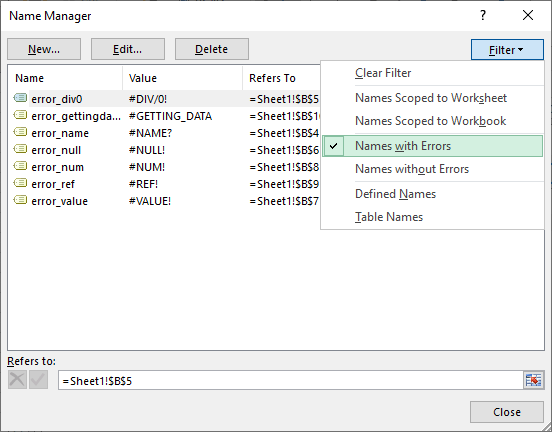## Color coded parentheses in Excel for easier formula creation

It is very easy to lose track when creating complex formulas in Excel. Since functions use parenthesis to wrap their arguments, you may end up in a position that you do not know which parenthesis belongs to which function. In this kind of situations, you need to pay...## How to how to debug an Excel formula using the F9 key

Debugging formulas in Excel can be intimidating. The process becomes harder if you are trying to calculate and also remember the values of each part of the formula. Fortunately, the F9 key is an easy and quick way to break a formula down into pieces. The F9 key...## How to analyze complex formulas with the Excel Evaluate formula feature

Are extremely long, formulas nested arguments intimidating you? The Evaluate Formula feature can help you untangle these complex formulas! This tool essentially solves complex formulas step by step. In this guide, we’re going to show you how to step through...## How to Make Excel Change Scope of Named Range

Named ranges have a property named “scope”. Usually, you do not need to adjust this property to use named ranges in Excel. On the other hand, it can become very useful if you have a number of sheets that are identical. The scope concept allows you to use...## How to apply named ranges to existing formulas in Excel

The use of named ranges is a very powerful tool for creating references in Excel. In addition to making formulas easier to read, you don’t have to remember the exact cell and range references when writing formulas. If you have already been using named ranges,...## How to use Wildcard criteria in Excel formulas

Wildcard is a term for a special kind of a character that can represent one or more “unknown” characters, and Excel has a wildcard character support. You can use wildcards for filtering, searching, or inside the formulas. In this guide, we’re going...## How to simplify Nested IF statements

Nested IF statements are useful for creating more complicated scenarios in Excel. However, things can get quickly out of hand as the number of conditions increase. For example, five conditions means at least four IF functions, hence lots of parenthesis to deal with....## How to Make Excel Remove Named Range Containing Errors

Managing and removing named ranges in Excel can be a challenging task, as you would have to do this one by one for each field. In this guide, we’re going to show you how to make Excel remove named range containing errors. Download Workbook Filtering named ranges with...